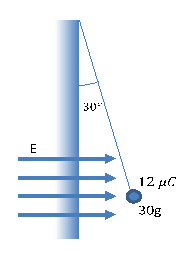# Problem: A pendulum has a charged mass at the end of it, as shown in the figure below. If it is in equilibrium, what is the magnitude of the electric field?

🤓 Based on our data, we think this question is relevant for Professor Khan's class at VT.

###### Problem Details

A pendulum has a charged mass at the end of it, as shown in the figure below. If it is in equilibrium, what is the magnitude of the electric field?What scientific concept do you need to know in order to solve this problem?

Our tutors have indicated that to solve this problem you will need to apply the Electric Field concept. You can view video lessons to learn Electric Field. Or if you need more Electric Field practice, you can also practice Electric Field practice problems.

What is the difficulty of this problem?

Our tutors rated the difficulty ofA pendulum has a charged mass at the end of it, as shown in ...as high difficulty.

How long does this problem take to solve?

Our expert Physics tutor, Juan took 4 minutes and 28 seconds to solve this problem. You can follow their steps in the video explanation above.

What professor is this problem relevant for?

Based on our data, we think this problem is relevant for Professor Khan's class at VT.